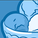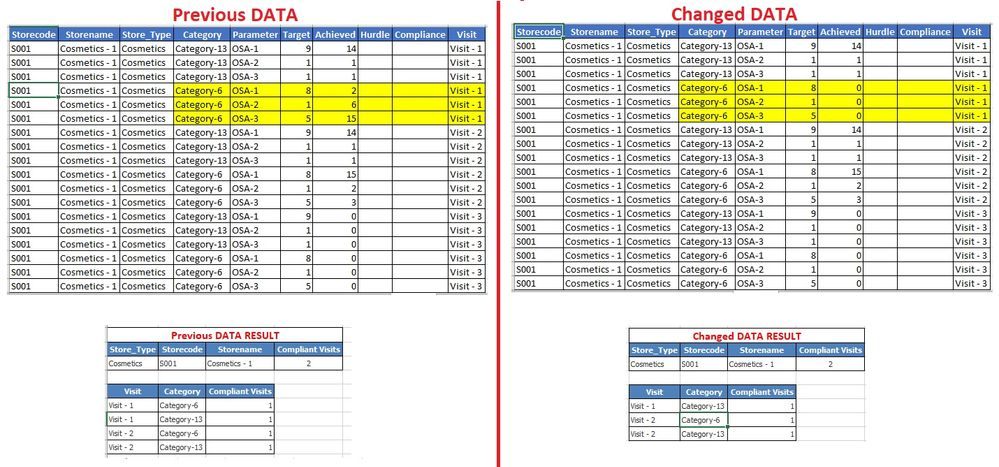# QlikView App Dev

Discussion Board for collaboration related to QlikView App Development.

Announcements
On May 18th at 10AM EDT we will answer your QlikView questions live. REGISTER
cancel
Showing results for
Did you mean:Contributor II

## Nested Sum(Aggr(Sum())) and match with Criteria

Hi All,

I have a scenario of calculating a Visit as Compliant based on the Categories (if matching criteria). But i am unable to do a nested sum or overall count on below expression. Can you please guide and help me out.

I will be very thankful to you.

CRITERIA:

Category-6 : 14

Category-13: 10

TRIED EXPRESSION:

if(
sum(aggr(sum({<Parameter={'OSA-1','OSA-2','OSA-3'},Store_Type={'Cosmetics'},Category={'Category-6'}>} Achieved), Storecode,Visit,Category))
>=14,1,0)
+
if(
sum(aggr(sum({<Parameter={'OSA-1','OSA-2','OSA-3'},Store_Type={'Cosmetics'},Category={'Category-13'}>} Achieved), Storecode,Visit,Category))
>=10,1,0)

DATA and RESULT I AM GETTING vs EXPECTED:Labels (3)

• ### SUM IFS

1 Solution

Accepted SolutionsMVP

try below

``````count(distinct aggr(if(sum(total<Store_Type,Category>aggr(if(Sum({<Parameter = {'OSA-1','OSA-2','OSA-3'}, Store_Type = {'Cosmetics'},Category = {'Category-6'}>} Achieved) >=14,1),Category,Store_Type))=1,Visit),Category,Store_Type,Parameter))
+
count(distinct aggr(if(sum(total<Store_Type,Category>aggr(if(Sum({<Parameter = {'OSA-1','OSA-2','OSA-3'}, Store_Type ={'Cosmetics'}, Category = {'Category-13'}>} Achieved) >=10,1),Category,Store_Type))=1,Visit),Category,Store_Type,Parameter))``````
5 RepliesMVP

May be try this

``````Sum(Aggr(
If(
Sum({<Parameter = {'OSA-1','OSA-2','OSA-3'}, Store_Type = {'Cosmetics'},
Category = {'Category-6'}>} Achieved) >= 14
or
Sum({<Parameter = {'OSA-1','OSA-2','OSA-3'}, Store_Type ={'Cosmetics'}, Category = {'Category-13'}>} Achieved)
, Visit)
, Visit, Category))``````Contributor II
Author

i tried your provided solution but it is not returning any result.

Can you please suggest some more option?MVP

Sorry my bad, can you try this

``````Count(DISTINCT Aggr(
If(
Sum({<Parameter = {'OSA-1','OSA-2','OSA-3'}, Store_Type = {'Cosmetics'},
Category = {'Category-6'}>} Achieved) >= 14
or
Sum({<Parameter = {'OSA-1','OSA-2','OSA-3'}, Store_Type ={'Cosmetics'}, Category = {'Category-13'}>} Achieved)
, Visit)
, Visit, Category))``````Contributor II
Author

The problem is that it is not checking if both the categories are compliant for the visit.

After changing the Data for one Category of Visit-1 but the expression still counting that Visit as Compliant.

It should be excluded.

MODIFIED EXPRESSION:

=Count(DISTINCT
Aggr(
If(
Sum({<Parameter = {'OSA-1','OSA-2','OSA-3'}, Store_Type = {'Cosmetics'},Category = {'Category-6'}>} Achieved) >= 14
or
Sum({<Parameter = {'OSA-1','OSA-2','OSA-3'}, Store_Type ={'Cosmetics'}, Category = {'Category-13'}>} Achieved) >= 10
,Visit)
,Visit, Category)
)

Changed DATA still counting Visit-1 as Compliant based on only 1 Category while it should check both Categories:MVP

try below

``````count(distinct aggr(if(sum(total<Store_Type,Category>aggr(if(Sum({<Parameter = {'OSA-1','OSA-2','OSA-3'}, Store_Type = {'Cosmetics'},Category = {'Category-6'}>} Achieved) >=14,1),Category,Store_Type))=1,Visit),Category,Store_Type,Parameter))
+
count(distinct aggr(if(sum(total<Store_Type,Category>aggr(if(Sum({<Parameter = {'OSA-1','OSA-2','OSA-3'}, Store_Type ={'Cosmetics'}, Category = {'Category-13'}>} Achieved) >=10,1),Category,Store_Type))=1,Visit),Category,Store_Type,Parameter))``````